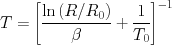# Chapter 9 Thermistor

This module provides a function to calculate the temperature of a thermistor using an exponential model for NTC (negative temperature coefficient)thermistors.

## 9.1  Usage

To use the thermistor function, use this:

  #include <thermistor.h>


## 9.2  Functions

#### float thermistor_t2_at_r2(float beta, float t1, float r_t1, float r_t2)function

This function uses an exponential model for NTC thermistors to approximate the thermistor’s temperature from its resistance. This function returns a thermistor’s temperature given the thermistor’s β value, beta, the nominal resistance, r_t1, at the nominal temperature, t1, and the measured resistance, r_t2. The temperatures must be in an absolute temperature scale; e.g., Kelvin. The equation used is:## 9.3  Caveat

Generally, this model is not as accurate as a table lookup can be. The measured temperature should fall within the range that the β value was calculated for, and if you have a choice of β values, you should choose the β value calculated over the smallest range that still suits your expected temperature range.

Also, this module uses floating calculations and log() to perform its calculation. The floating-point library is large and floating point calculations can be relatively slow.